Get instant live expert help with Excel or Google Sheets“My Excelchat expert helped me in less than 20 minutes, saving me what would have been 5 hours of work!”

#### Post your problem and you’ll get Expert help in seconds.

Your message must be at least 40 characters
Our professional Expert are available now. Your privacy is guaranteed.

# How to Get the Last Name from Name

We can extract the last name from the full name of an individual in a cell by using a combination of the following functions: RIGHT, LEN, FIND, and SUBSTITUTE. The steps below will walk through the process.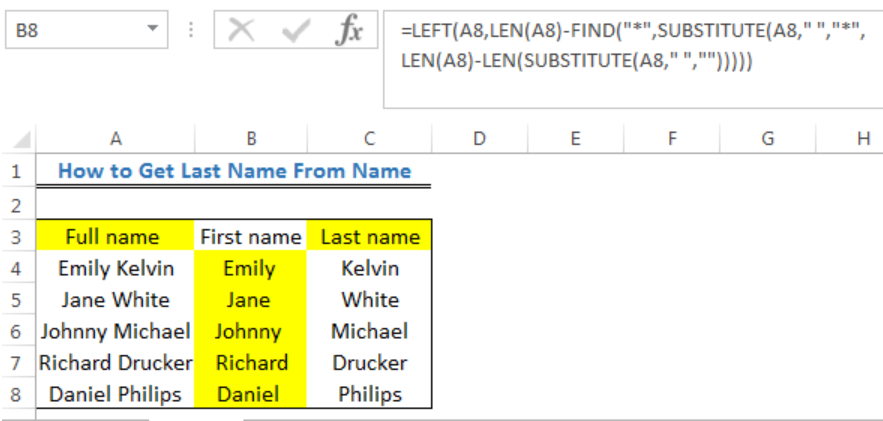Figure 1: How to Get Last Name from Name

## Formula

`=RIGHT(name,LEN(name)-FIND("*",SUBSTITUTE(name," ","*",LEN(name)-LEN(SUBSTITUTE(name," ","")))))`

Note that the name is a full name that contains a blank space between the first name and the last name.

## Setting up the Data

• We will set up the data by inserting the full names in Column A.
• Column B will contain the First name and Column C will contain the Last name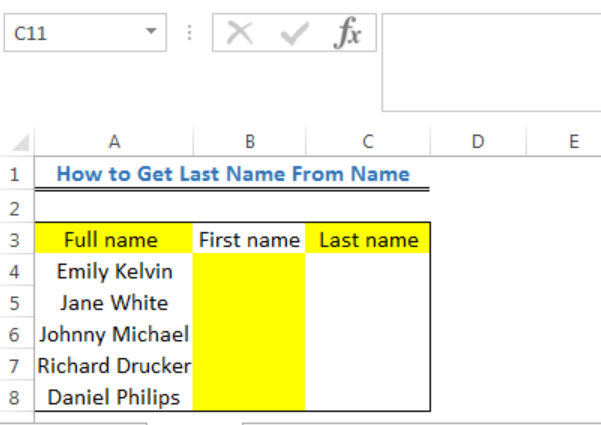Figure 2: Setting up the Data to Get the Last Name from Name

## Get the Last Name from Name

• We will click on Cell C4
• We will insert the formula below

`=RIGHT(A4,LEN(A4)-FIND("*",SUBSTITUTE(A4," ","*",LEN(A4)-LEN(SUBSTITUTE(A4," ","")))))`

• We will press the enter key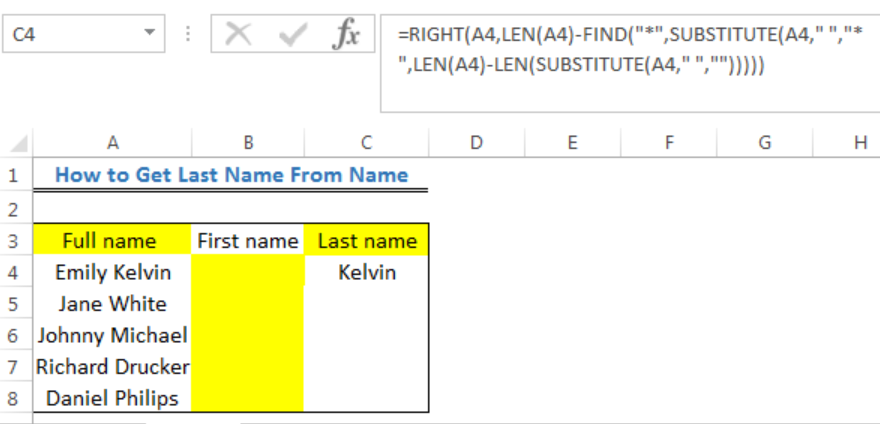Figure 3: Inputting the formula to Get the Last Name from Full Name in Cell A4

• We will use the drop-down feature to drag down and get the last names of the remaining cells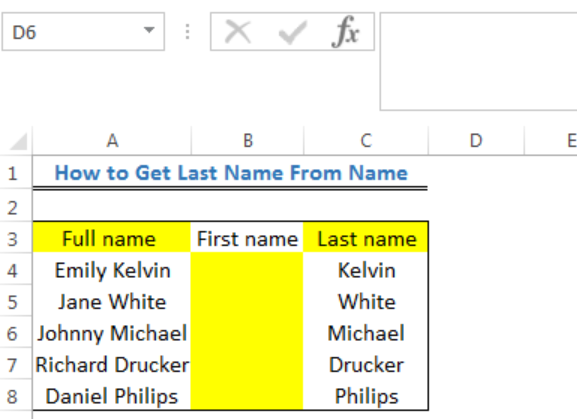Figure 4: Result of the Last Name from Full Names

## Get the First Name from Name

We can get the first names by changing RIGHT in the formula to LEFT.

We will input the formula below into Cell B4, press enter and drag down to get the values for the remaining cells.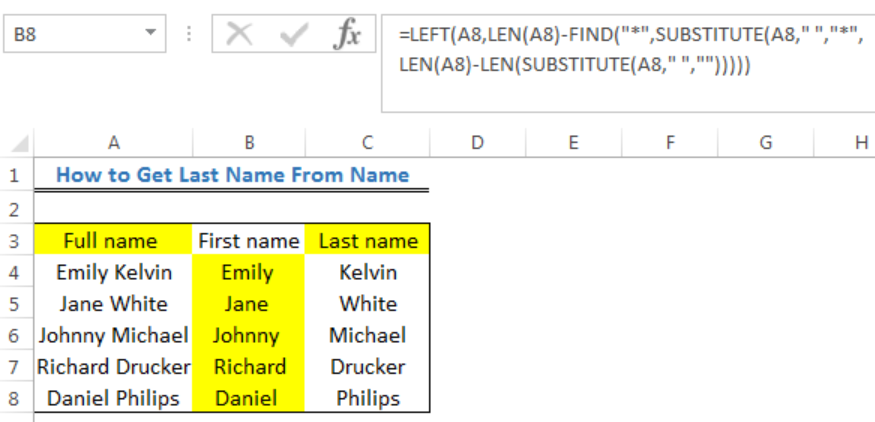Figure 5: Result of the First Name from Full Names

## Explanation

Formula: `=RIGHT(A4,LEN(A4)-FIND("*",SUBSTITUTE(A4," ","*",LEN(A4)-LEN(SUBSTITUTE(A4," ","")))))`

The RIGHT function is used to extract characters starting from the right. The formula uses an asterisk (“*”) to replace the last space in the full name. The FIND function is used to locate the exact position of the asterisk. This enables the RIGHT function to know where to stop extracting values from the right.

The SUBSTITUTE function performs the job of replacing the space in the name with the asterisk. This is based on the section of the formula below:

`=SUBSTITUTE(A4," ","*",LEN(A4)-LEN(SUBSTITUTE(A4," ","")))))`

The SUBSTITUTE function below replaces the space in A4 with no space. From this part of the function, we will observe that the asterisk is absent.

`=SUBSTITUTE(A4," ","") = EmilyKelvin`

The LEN function below calculates the number of spaces. This is indicated by the inverted commas which pulls the texts in the cell together.

`=LEN(A4)-LEN(SUBSTITUTE(A4," ","")))))`

LEN A4 is the total number of characters including the space in Cell A4. That is 12.

`LEN(SUBSTITUTE(A4," ",""))))) `is the total number of characters excluding the space in Cell A4. That is 11.

As a result, the number of spaces in the name in Cell A4 is 1.

The SUBSTITUTE function below can now be like this IN THE RIGHT:

`=SUBSTITUTE(A4," ","*",  = SUBSTITUTE(A4," ","*",1)`

The SUBSTITUTE Function establishes that there is only one space and identifies the position of the space.

The FIND function locates the asterisk and returns the position of the asterisk in the name.

`=FIND(“*”,Emily*Kelvin)`

The result is 6 because “*” is in the 6th position. It is then subtracted by the length of the name by:

`=LEN(A4)-6`

`12 – 6 = 6`

The number 6 is then used by RIGHT function:

`=RIGHT(A4,6)`

This returns the result as Kelvin.

## Note

• Inconsistent spacing poses as a common error that can be fixed with the TRIM function

## Instant Connection to an Expert through our Excelchat Service

Most of the time, the problem you will need to solve will be more complex than a simple application of a formula or function. If you want to save hours of research and frustration, try our live Excelchat service! Our Excel Experts are available 24/7 to answer any Excel question you may have. We guarantee a connection within 30 seconds and a customized solution within 20 minutes.

### Did this post not answer your question? Get a solution from connecting with the expert.Another blog reader asked this question today on Excelchat:
Solution examplesI have text and number in multiple cells (Ex: Cell 1: 4S, Cell 2: 3.5A, Cell 3: 8O) and want to sum the number values only (Ex: 4 + 3.5 + 8). How can I do this?
Solved by O. A. in 60 minsWhat is the formula for splitting up one column text into 2 columns?
Solved by B. H. in 20 minsCan you re edit this formula for me =IFERROR(IF(LEN(MID("RHS",1,FIND("RHS",B4,1)))=1,LEFT(B4,3),MID("RHS",1,FIND("RHS",B4,1))),MID("LHS",1,FIND("LHS",B4,1)))
Solved by S. L. in 40 minsI am having issues creating the one way data table to show the means and standard deviations when you change the proportion of portfolio 1. I have the mean formula and the standard deviation formula at the top and -1 through 1 down the left side. I highlight all of it and create a data table. I reference the "proportion of portfolio 1" cell in the column input box but when the data table comes out it has all the same numbers all they way down the table.
Solved by G. Q. in 49 minsI need to create a formula that extracts the prefix from column A
Solved by I. Q. in 42 mins## Subscribe to Excelchat.coAnother blog reader asked this question today on Excelchat: